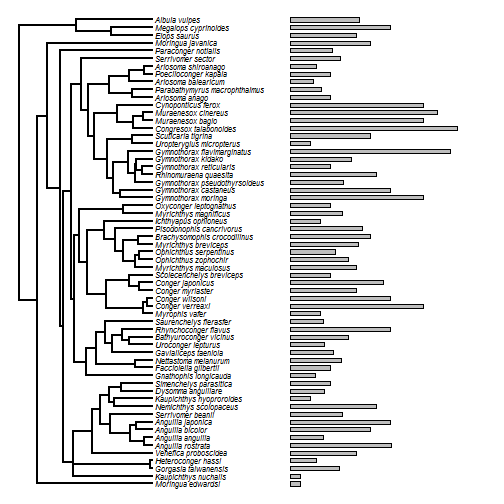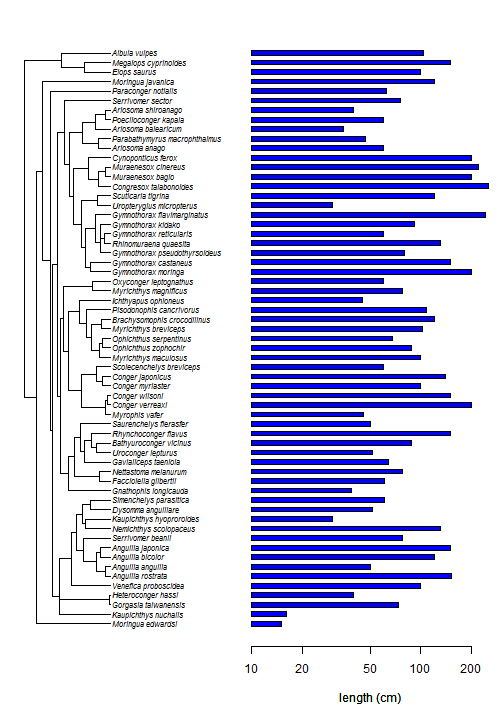## Thursday, July 21, 2016

### More on showing a barplot next to a plotted tree

I just added a new function to phytools - `plotTree.barplot` - to reproduce the visualization shown in a previous recent post.

The idea is to put a barplot next to a plotted tree, but in which the bars line up nicely with the tips.

There already exists a function in phytools to do this kind of visual. It is quite flexible, but it works by just drawing rectangles in the plotting device where we have already drawn a tree. To do this, the user needs to set a scale for the rectangles (the units of measure for our trait are not usually scaled to the edges of the phylogeny), and there is not an obvious way to include an axis.

Here is a demo of that function, using the data & tree that I employed in my prior recent demo:

``````library(phytools)
plotTree.wBars(eel.tree,bsize,scale=0.5,tip.label=TRUE,fsize=0.7)
``````The new implementation results in a highly similar plot - but instead of attempting to plot the bars & the tree in the same device, it opens a plot array using `par()\$mfrow` and then prints the tree & bar plot to different subplots. This means that they are no longer required to be rendered to the same scale, and it is very easy to include an axis or other labels on the bar plot.

To accomplish this & still permit the user to pass whatever arguments they wanted to `plotTree` and `barplot`, I have made use of the function `do.call`, which executes a function with a list of arguments passed to it. That means that arguments that the user wants to send to each of these functions needs to be supplied in two lists: `args.plotTree` (to be passed to `plotTree`), and `args.barplot`.

First, here is the function code:

``````plotTree.barplot<-function(tree,x,args.plotTree=list(),
args.barplot=list()){
cw<-reorder(tree)
args.barplot\$height<-x[cw\$tip.label]
args.barplot\$plot<-FALSE
args.barplot\$horiz<-TRUE
args.barplot\$axes<-FALSE
args.barplot\$names.arg<-""
if(is.null(args.barplot\$space)) args.barplot\$space<-0.7
if(is.null(args.barplot\$mar))
args.barplot\$mar<-c(5.1,0,2.1,1.1)
else args.barplot\$mar<-0.1
args.plotTree\$tips<-setNames(do.call(barplot,args.barplot)[,1],
cw\$tip.label)
args.barplot\$plot<-TRUE
args.barplot\$ylim<-range(args.plotTree\$tips)
args.plotTree\$tree<-cw
if(is.null(args.plotTree\$mar))
args.plotTree\$mar<-c(5.1,1.1,2.1,0)
else {
args.plotTree\$mar<-0.1
}
if(args.plotTree\$mar!=args.barplot\$mar)
args.plotTree\$mar<-args.barplot\$mar
if(args.plotTree\$mar!=args.barplot\$mar)
args.plotTree\$mar<-args.barplot\$mar
if(is.null(args.plotTree\$ftype)) args.plotTree\$ftype<-"i"
if(is.null(args.plotTree\$lwd)) args.plotTree\$lwd<-1
par(mfrow=c(1,2))
do.call(plotTree,args.plotTree)
par(mar=args.barplot\$mar)
obj<-do.call(barplot,args.barplot)
axis(1)
if(!is.null(args.barplot\$xlab)) title(xlab=args.barplot\$xlab)
else title(xlab="x")
invisible(obj)
}
``````

Now here is an example:

``````plotTree.barplot(eel.tree,bsize,list(fsize=0.7),
list(col="blue",space=1,log="x",
xlim=c(10,max(bsize)),xlab="length (cm)"))
``````You get the idea.

1.This comment has been removed by the author.

1.This comment has been removed by the author.

2.Hello Liam, thanks to share this post, It´s seems very useful. I’m wondering if there is a way to plot two data bar lines, one on top of the other (these are for two independent data set) next to the same phylogeny and differentiate them with different colors.
Cheers,

1.Hi Carlos. This is possible, but you need to install the development version of phytools from GitHub. More information is here.

All the best, Liam

2.Thank you Liam, it was very helpfull!
- Carlos

3.Hello Liam, thanks for the post and all the information. I wonder if there is way of plotting different tip labels: For example, some in bold and some italics. It would be really helpful. Cheers, Julia

1.maybe I didn´t explained myself properly. I mean, if it´s possible to print in the same plot, some tip labels in bold and some tip labels in italics.

2.This can be done for trees plotted with plot.phylo by just provided the font types as a vector. Right now it is not possible with phytools but this seems like a good idea so I'm going to add it.

4.Very neat tool to plot barchart next to a tree. Is there a way to color the branches, and also add an axis and axis title to the tree?

using the following code did nothing to add color the the branch:

for(i in 1:length(tt)) tree<-paintSubTree(tree,node=i,state=tt[i],stem=TRUE)
colors<-setNames(c("black","red","blue"),c("KB","PM","KW"))

1.